# Maximal Update Parametrization (μP) and Hyperparameter Transfer (μTransfer)

In Tensor Programs V: Tuning Large Neural Networks via Zero-Shot Hyperparameter Transfer, we show that optimal hyperparameters become stable across neural network sizes when we parametrize the model in maximal update parametrization (μP). This can be used to tune extremely large neural networks such as large pretrained transformers, as we have done in our work. More generally, μP reduces the fragility and uncertainty when transitioning from exploration to scaling up, which are not often talked about explicitly in the deep learning literature.Figure above: Training loss against learning rate on Transformers of varying `d_model` trained with Adam.

μP turns out to be the unique "natural" parametrization that has this hyperparameter stability property across width, as empirically verified in the gif below on MLPs trained with SGD. Here, across time, we interpolate between PyTorch default and μP's learning rate and initialization scalings (right), and we scale up the width-256 model (log2(width)=8) to width 2^13 = 8192 using this interpolated scaling rule (left).This repo contains the source code for the `mup` package, our tool that makes the implementation of μP in Pytorch models effortless and less error-prone.

## Installation

``````pip install mup
``````

### Install From Source

Clone this repo, change to its directory, and do

``````pip install -r requirement.txt
pip install -e .
``````

## Basic Usage

```from mup import MuReadout, make_base_shapes, set_base_shapes, MuSGD, MuAdam

class MyModel(nn.Module):
def __init__(self, width, ...):
...
### In model definition, replace output layer with MuReadout
### If tying weights with an input nn.Embedding layer, do
...
def forward(self, ...):
...
### If using a transformer, make sure to use
###   1/d instead of 1/sqrt(d) attention scaling
# attention_scores = query @ key.T / d**0.5
attention_scores = query @ key.T * 8 / d
### We use 8/d instead of 1/d here to be backward compatible
###   with 1/d**0.5 when d=64, a common head dimension.
...

### Instantiate a base model
base_model = MyModel(width=1)
### Optionally, use `device='meta'` to avoid instantiating the parameters
### This requires you to pass the device flag down to all sub-modules
# base_model = MyModel(width=1, device='meta')
### Instantiate a "delta" model that differs from the base model
###   in all dimensions ("widths") that one wishes to scale.
### Here it's simple, but e.g., in a Transformer, you may want to scale
###   both nhead and dhead, so the delta model should differ in both.
delta_model = MyModel(width=2) # Optionally add the `device='meta'` to avoid instantiating

### Instantiate the target model (the model you actually want to train).
### This should be the same as the base model except
###   the widths could be potentially different.
### In particular, base_model and model should have the same depth.
model = MyModel(width=100)

### Set base shapes
### When `model` has same parameter shapes as `base_model`,
###   `model` behaves exactly the same as `base_model`
###   (which is in PyTorch's default parametrization).
###   This provides backward compatibility at this particular model size.
###   Otherwise, `model`'s init and LR are scaled by μP.
### IMPORTANT: this should be called as soon as possible,
###   before re-initialization and optimizer definition.
set_base_shapes(model, base_model, delta=delta_model)

### Alternatively, one can save the base model shapes in a file
# make_base_shapes(base_model, delta_model, filename)
### and later set base shapes directly from the filename
# set_base_shapes(model, filename)
### This is useful when one cannot fit both
###   base_model and model in memory at the same time

### Replace your custom init, if any
for param in model.parameters():
### If initializing manually with fixed std or bounds,
### then replace with same function from mup.init
# torch.nn.init.uniform_(param, -0.1, 0.1)
mup.init.uniform_(param, -0.1, 0.1)
### Likewise, if using
###   `xavier_uniform_, xavier_normal_, kaiming_uniform_, kaiming_normal_`
### from `torch.nn.init`, replace with the same functions from `mup.init`

### Use the optimizers from `mup.optim` instead of `torch.optim`
# optimizer = torch.optim.SGD(model.parameters(), lr=0.1)
optimizer = MuSGD(model.parameters(), lr=0.1)

### Then just train normally```

Note the base and delta models do not need to be trained --- we are only extracting parameter shape information from them. Therefore, optionally, we can avoid instantiating these potentially large models by passing `device='meta'` to their constructor. However, you need to make sure that the `device` flag is appropriately passed down to the constructor of all submodules. Of course, it'd be even better if PyTorch can do this automatically for any existing `nn.Module`. If you want to see this happen, please upvote this PyTorch issue.

## How `mup` Works Under the Hood

By invoking `set_base_shapes(model, ...)`, each parameter tensor `p` of `model` gets a `p.infshape` attribute that stores, for each of its dimensions, the corresponding base dimension and whether that dimension should be considered `infinite` (i.e. will be scaled up/down, e.g., `d_model` of a Transformer) or `finite` (i.e. will be fixed, e.g., vocabulary size). This information is used in the initializers and optimizers to automatically scale the parameters or learning rates to be compliant with μP. For example, the Adam learning rate of hidden weights `p` is calculated as `globalLR / p.infshape.width_mult()`, where `p.infshape.width_mult()` essentially calculates `fan_in / base_fan_in`.

## Current Limitations

• `set_base_shapes(model, ...)` assumes that `model` has just been randomly initialized in the standard way and rescales its parameters using the base shape information so the model is in μP.
• If you want data parallelism, please use `torch.nn.parallel.DistributedDataParallel` instead of `torch.nn.DataParallel`. This is because the latter removes the attributes the `mup` package adds to each parameter tensor of the model. Also, for performance, `pytorch` recommends the former anyway.
• We scale the learning rate according to μP explicitly by creating refined parameter groups from what is passed to the `mup` optimizer and by manipulating the `lr` attribute in those groups. This is compatible with PyTorch's learning rate schedulers. However, if you roll your own, make sure the scheduler sets the learning rate relative to what is currently in the refined parameter groups. The following is an example of what not to do and what is OK:
```optimizer = mup.MuAdam(model.parameters(), lr=1e-3)
for pg in optimizer.param_groups:
# what NOT to do: setting learning rate absolutely
# pg['lr'] = 1e-3 * 2
# what is an OK alternative: setting it relatively
pg['lr'] *= 2```
• By default, any parameter matrix that has 2 "infinite" dimensions (i.e. dimensions that are different from base dimensions) are considered by `mup` to have shape (fan_out, fan_in), i.e., in the forward pass, this matrix multiplies its input on the right. This is the case with all `nn.Linear` weights from pytorch. If you have a custom parameter, say `W`, that violates this convention, you can manually set `W.infshape.main_idx = 0; W.infshape.main = W.infshape` to let `mup` know that its shape corresponds to (fan_in, fan_out). A similar discussion applies if you have a parameter tensor with many dimensions but exactly 2 "infinite" dimensions, for which the first is fan_in and the second is fan_out.
• Currently, `torch.save` does not save the `infshape` objects attached to each parameter tensor. Before this is fixed, you would have to set base shape manually after loading a model checkpoint like so:
```model = torch.load('my/model/path.pt')
# Important: note the flag `rescale_params=False`!
set_base_shapes(model, 'my/base/shape/path.bsh', rescale_params=False)```

(`set_base_shapes` by default rescales the parameters of `model`, assuming it's freshly initialized by PyTorch, to be consistent with μP. The `rescale_params=False` flag turns off this behavior.)

## Checking Correctness of Parametrization

### Coord Check

Just like gradient checking is a simple way of verifying the correctness of an autograd implementation, coordinate checking is a simple way to verify you have implemented μP correctly: calculate the average size (which we denote in the y-axis below by `l1`) of the coordinates of each activation vector in, and output of, the model, for a few steps of training and a few different widths. If implemented correctly, then we shall see this `l1` stable over many widths; otherwise, the `l1` can blow up or shrink to 0 with width. (We are essentially checking desideratum 1 described below.) (The `l1` calculates `x.abs().mean()` for each activation vector `x` and is just one measure of the "average size" of `x`'s entries; one can also use analogously defined `l2`, `l4`, etc, though they may exhibit greater fluctuation with random seeds.)

For example, in the following, we plot `width` vs `l1` for 2 steps of training, where t=1 means at initialization, before any gradient update. Each curve corresponds to an (pre-)activation vector of a layer or the output of the network. The first set of 3 plots shows an MLP in standard parametrization (SP), trained by adam. We see after 1 step of update, activation/output `l1` are exploding with width. This means SP is "incorrect."We now do the same for an MLP in maximal update parametrization (μP) (including using `mup.optim.MuAdam` instead of `torch.optim.Adam`). In contrast to the above, all curves stay horizontal, indicating that μP is implemented correctly.We call this way of checking implementation correctness a coord check, short for "coordinate check."

### Making Your Own Coord Check Plots

We provide an easy way to implement this check via functions in the `mup.coord_check` module. The workflow typically looks like the following.

```from mup.coord_check import get_coord_data, plot_coord_data
# construct a dictionary of lazy μP models with differing widths
def lazy_model(width):
# `set_base_shapes` returns the model
return lambda: set_base_shapes(MyMuModel(width), 'my/base/shape/path.bsh')
# Note: any custom initialization with `mup.init` would need to
# be done inside the lambda as well
models = {64: lazy_model(64), ..., 1024: lazy_model(1024)}
# make a dataloader with small batch size/seq len
#   just for testing
# record data from the model activations over a few steps of training
# this returns a pandas dataframe
# This saves the coord check plots to filename.
plot_coord_data(df, save_to=filename)
# If you are in jupyter notebook, you can also do
#   `plt.show()`
# to show the plot```

For example, the `mup.coord_check.example_plot_coord_check` function is implemented this way for toy MLP and CNN models.

If you see the curves blow up or shrink to 0 with width after a few steps of training, then there's a bug in your μP implementation (did you forget to vary some dimension, like `d_ffn`, in the delta model?). If instead you see the curves converge to the right, then most likely your implementation is correct. However, there are two typical exceptions to this; the following can shrink to 0 at initialization in μP (at a 1/sqrt(width) rate):

• the network output
• the attention logits in a Transformer

These are transient, and after a few steps their curves should be roughly flat. Nevertheless, to remove the discrepancy at init, we recommend

• initializing the output layer (should be a `MuReadout` instance) weights to be 0 via the `readout_zero_init=True` option and
• initializing the query matrix in a Transformer to 0 (this has to be done manually). If symmetry-breaking is desired in the attention logits at init, initialize the (relative) position biases with nonzero variance.

#### Tips for Coord Check

• Use a large learning rate (larger than you'd use for actual training). This would emphasize any potential exploding coordinates issue, which could be hidden by the initialization if the learning rate is too small.
• If you reuse a module multiple times in the forward pass, then `mup.get_coord_data` will only record the statistics from the last usage. In this case, for testing purposes, one can wrap different usages with `nn.Identity` modules of different names to distinguish them.

### Wider is Always BetterAnother sign that μP has not been implemented correctly is if going wider does worse (on training loss) after some width, at some point during training. The figure above illustrates this in a collection of training curves: (left) the correct implementation should always see performance improve with width, at any point in training; (middle) if you used standard parametrization (SP), sometimes you may see performance improve with width up to some point and then suddenly it becomes worse with wider models; (right) or you may immediately see worsening performance even for narrow models.

## Examples

See the `MLP`, `Transformer`, and `ResNet` folders inside `examples/` as well as the tests in `mup/test` for examples. People familiar with Huggingface Transformers may also find the `examples/mutransformers` submodule instructive (obtained via `git submodule update --init`), which is also available standalone at https://github.com/microsoft/mutransformers.

## Native Integration With Huggingface

Frustrated that your Huggingface Transformer breaks when you scale up? Want to tune hyperparameters for your large mult-GPU Huggingface Transformer on a single GPU, right out the box? If so, please upvote this github issue!

## Running Tests

To run tests, do

`python -m mup.test`

## The Basic Math

μP is designed so as to satisfy the following desiderata:

At any time during training

1. Every (pre)activation vector in a network should have Θ(1)-sized coordinates
2. Neural network output should be O(1).
3. All parameters should be updated as much as possible (in terms of scaling in width) without leading to divergence

It turns out these desiderata uniquely single out μP. To derive μP from them, one needs to carefully consider how the coordinate size of a vector Av, resulting from a square matrix A multiplying vector v, depends on those of A and v, when A and v are "correlated". Here you can think of A as weights and v as an activation vector. This in turn depends on what kind of matrix is A and what kind of vector is v. In the context of training a wide neural network, it turns out we only need to consider vectors that has approximately iid coordinates, and two kinds of matrices: 1) those that look like outer products of such vectors, and 2) random iid matrices. Those of type 1 cover things like weight gradients; those of type 2 cover things like weight initialization. Then, if A and v both have entry size Θ(1) and they are correlated in ways that arise naturally during training, then we have the following table.

outer product A (type 1) iid A (type 2)
Entry size of Av Θ(n) Θ(sqrt(n))

Given this table, one can then trace the forward and backward computation of a network to derive μP straightforwardly.

See our blog post for a gentle primer and our paper for details.

## Contributing

This project welcomes contributions and suggestions. Most contributions require you to agree to a Contributor License Agreement (CLA) declaring that you have the right to, and actually do, grant us the rights to use your contribution. For details, visit https://cla.opensource.microsoft.com.

When you submit a pull request, a CLA bot will automatically determine whether you need to provide a CLA and decorate the PR appropriately (e.g., status check, comment). Simply follow the instructions provided by the bot. You will only need to do this once across all repos using our CLA.

This project has adopted the Microsoft Open Source Code of Conduct. For more information see the Code of Conduct FAQ or contact [email protected] with any additional questions or comments.

This project may contain trademarks or logos for projects, products, or services. Authorized use of Microsoft trademarks or logos is subject to and must follow Microsoft's Trademark & Brand Guidelines. Use of Microsoft trademarks or logos in modified versions of this project must not cause confusion or imply Microsoft sponsorship. Any use of third-party trademarks or logos are subject to those third-party's policies.

• #### Conv1D Coord check looks good (I think), but μTransfer does not seem to work?

Hi all, attaching the coord check plots and also a screen shot of the train loss and auprc plots. I used the Conv1D from the branch, but have also tried

I was looking at the conv plots from the examples and I noticed that one of the layers is constant across width, but after the first step is significantly smaller. Is that an issue?

Mup plot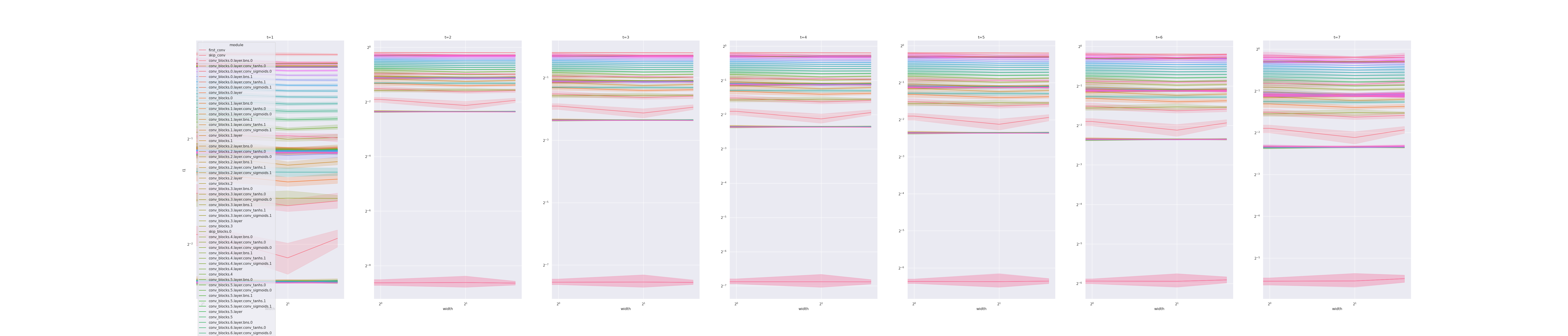Sp Plot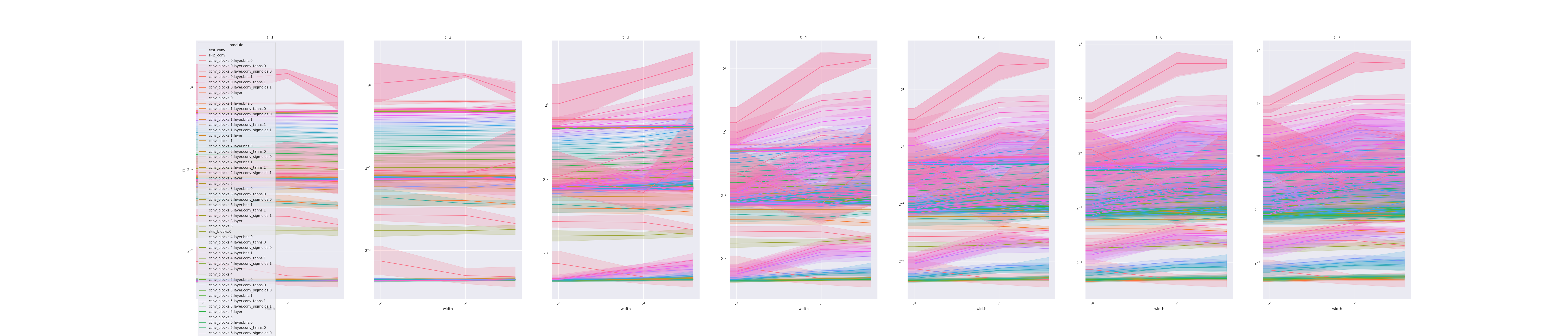Train Loss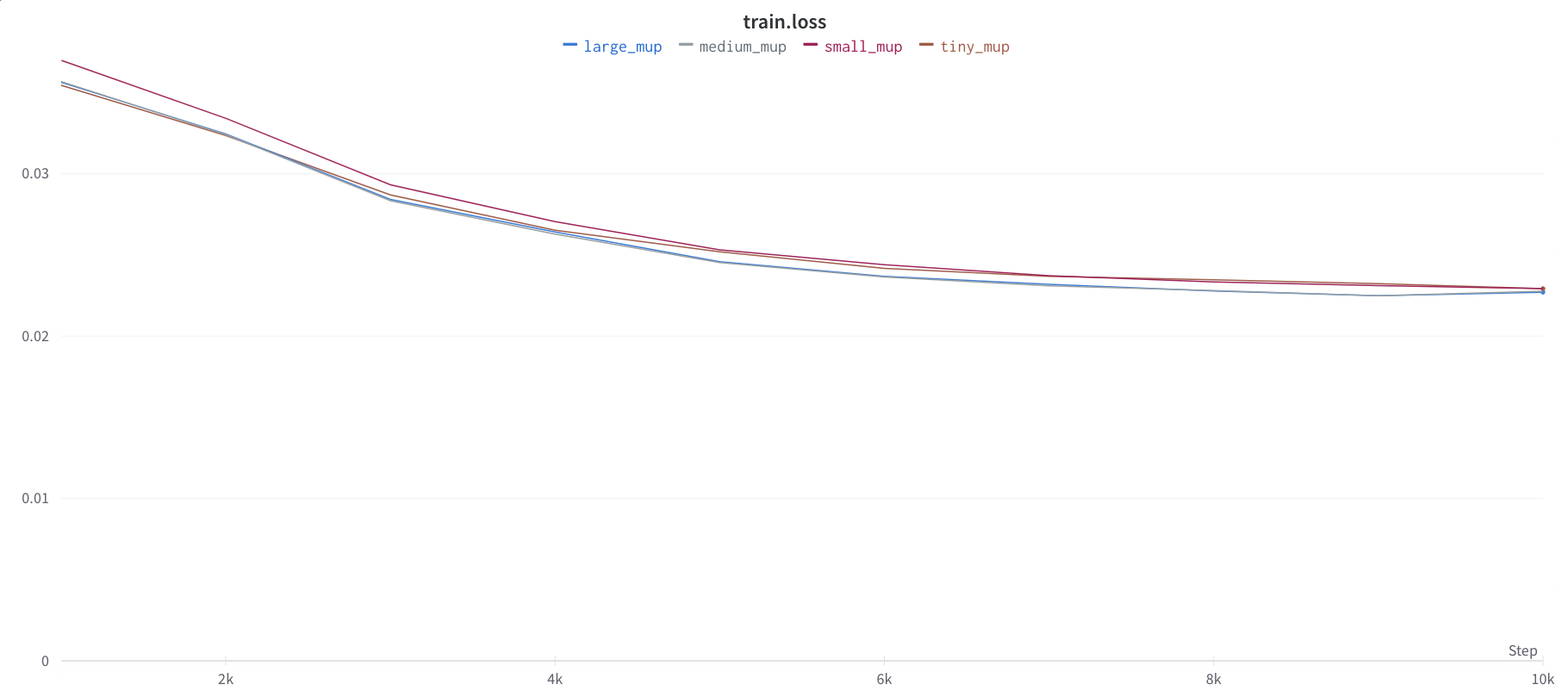Train AUPRC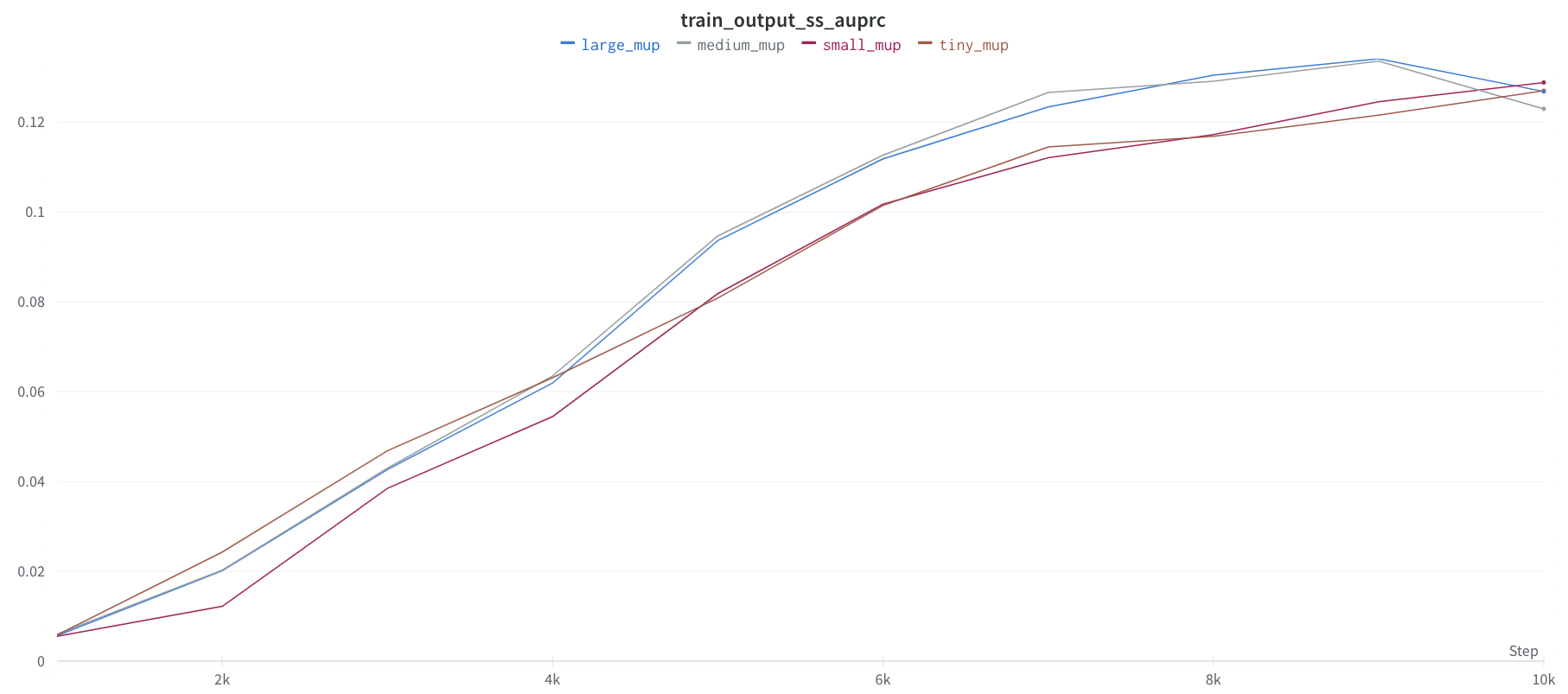I also tried this with a transformer based model and found similar results where the transferred HPs did not result in better performance. I can regenerate those plots if needed.

Is this expected? What can I do to fix this? Having `mup` work would be a huge unlock for us :D

opened by zanussbaum 20
• #### Coord-check for conv1d

I modified the muconv2d branch to get a conv1d variant of the output layer for mup, and I applied it to a shallow variant of a unet model I've been testing.

repo for model: https://github.com/bob80333/audio_bandwidth_extension fork of mup with conv1d: https://github.com/bob80333/mup/tree/muconv2d

Here's the coord-check results, they don't look quite a smooth as the paper but there's definitely a big difference between mup and no mup.

mup: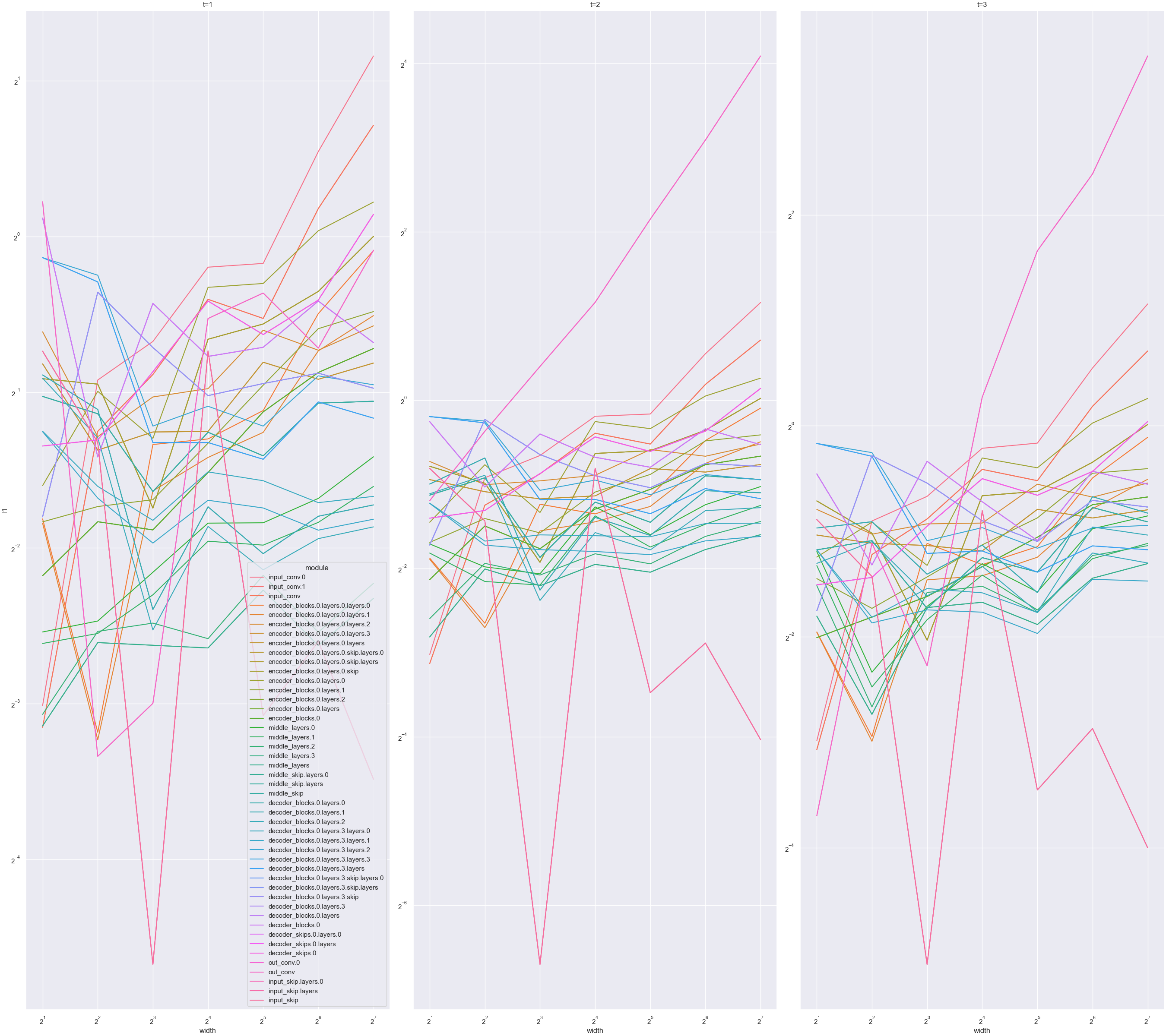no mup: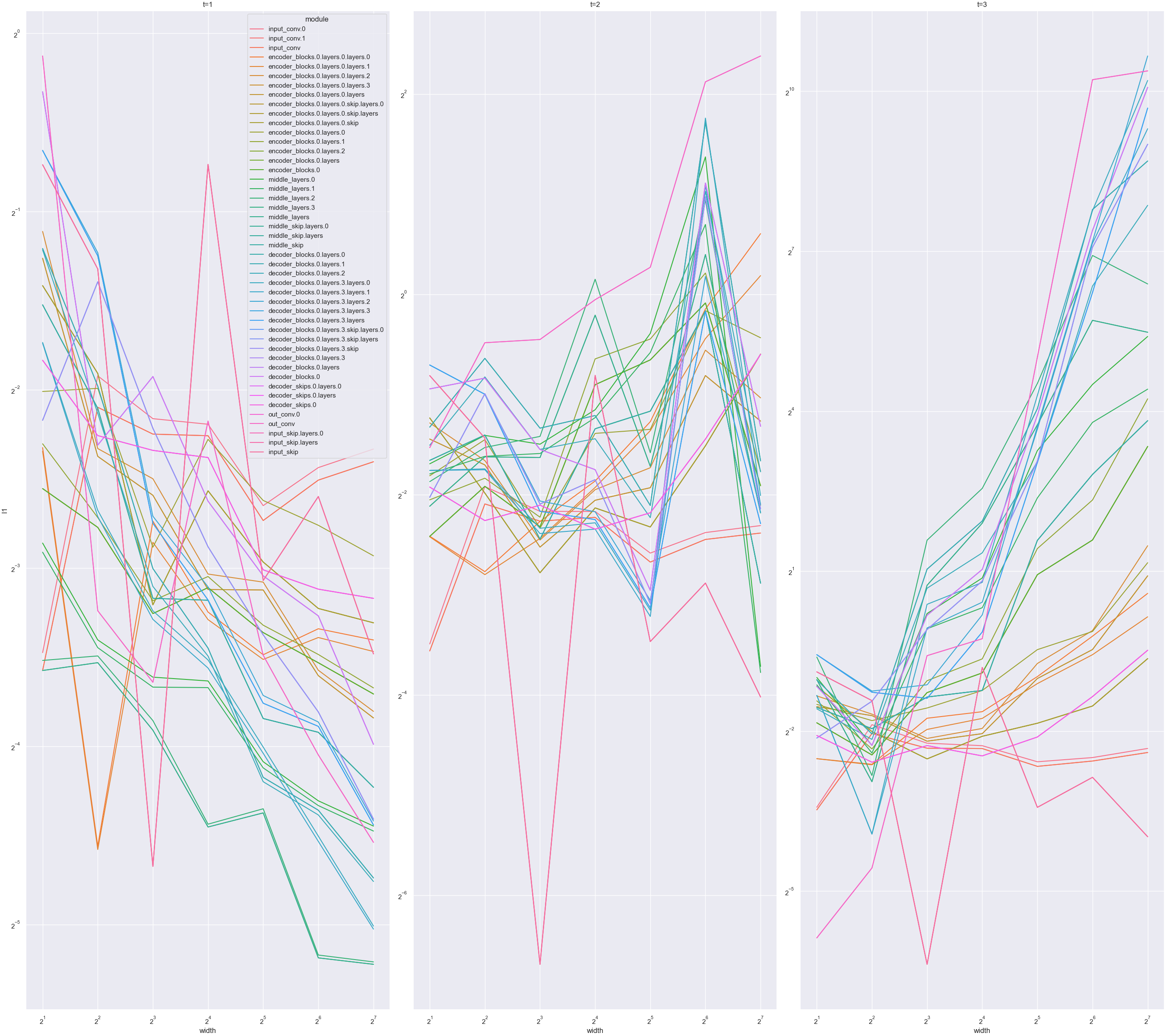Does this look about right for the coordinate check? The figures I saw in the example looked much smoother than this.

opened by bob80333 17
• #### MuP Coord Check not Working with Electra Style Model

I'm trying to use an Electra-Style model with µP but am not able to get a the coord plots to work correctly. Currently, I have `Readout` layers on both the `Discriminator` and `Generator`.

Creating coord checks for the `Discriminator` and `Generator` alone seem to work, but when combined the µP plot does not seem as expected.

Generator coord checks: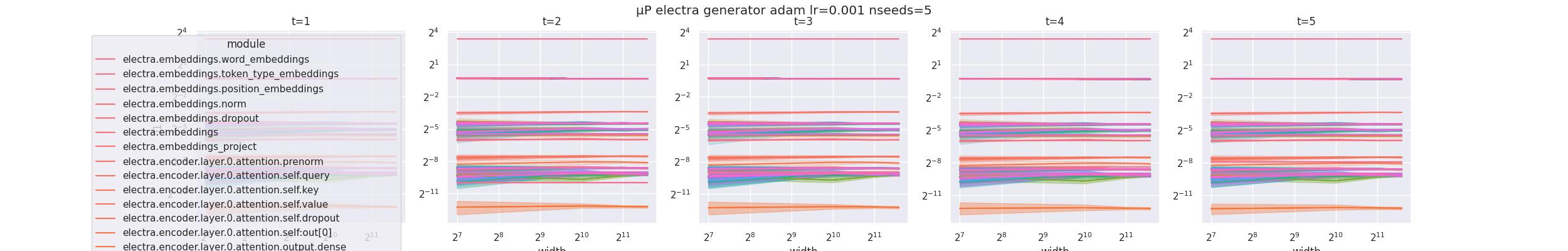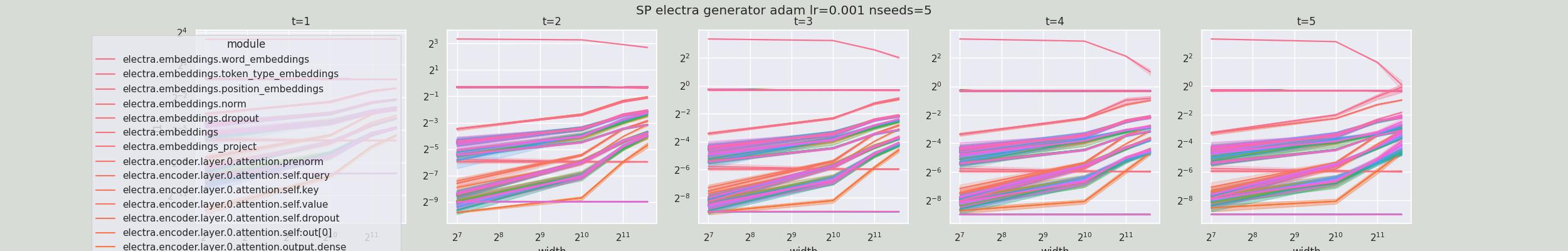Discriminator coord checks: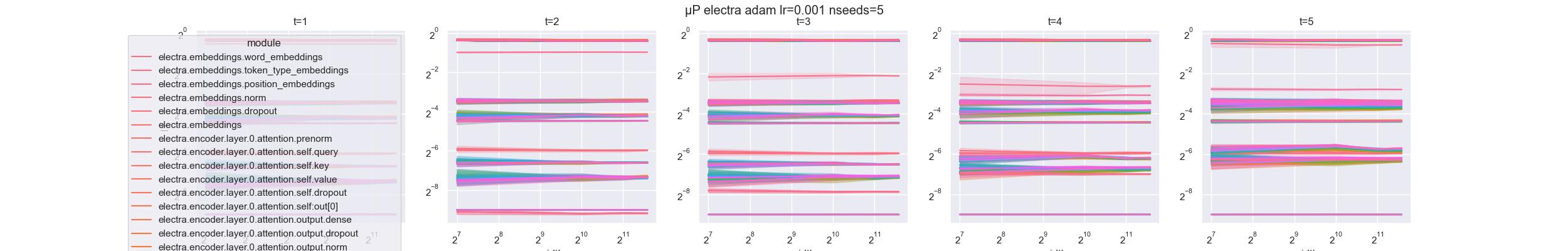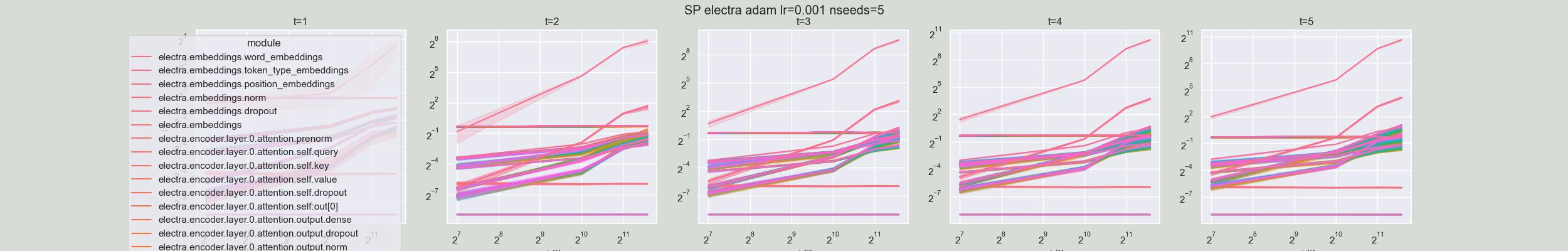Electra Model coord checks: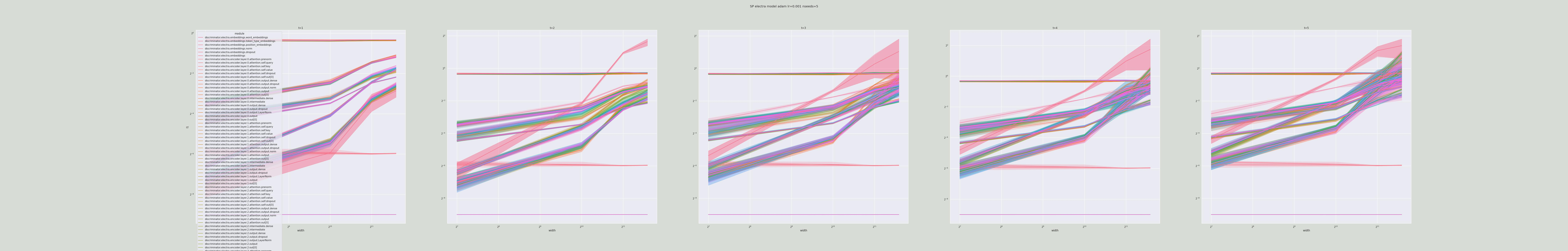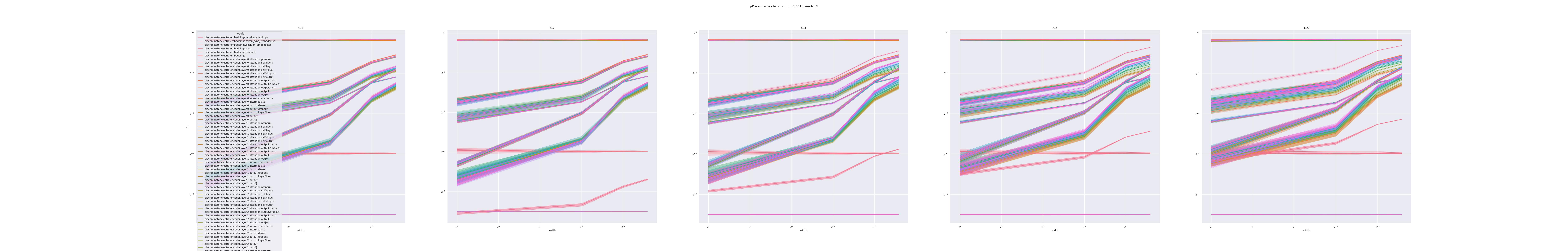Will µP not work for "multi-task" losses like here where the overall loss is a weighted sum of `mlm_loss` and `disc_loss`?

opened by zanussbaum 8
• #### Does mup work with model with Conv2D as output?

Hello, this project look great and the Github documentation is really good. Just wondering if mup would work with a model that have the last layer as nn.Conv2d instead of linear.

opened by BurguerJohn 6
• #### Issue in reproducing the training loss vs learning rates curve

Hi, First of all, thanks for sharing your work.

We tried to reproduce the expected behavior of muP, using ResNet18 and the CIFAR10, as provided in the main script of your repository. The idea was to launch a training, for multiple learning rates and width_mult, and get the minimum loss each time, as you did in your paper, to ensure that the best learning rate doesn't change with a different width_mult.

We modified a bit the main.py script, to skip the saving/loading of the base shape file, as follows:

``````'''Train CIFAR10 with PyTorch.'''
import argparse
import os
from time import gmtime, strftime
import numpy as np
import torch
import torch.nn as nn
import torch.optim as optim
import torchvision
import torchvision.transforms as transforms
from mup import MuAdam, MuSGD, get_shapes, set_base_shapes
from copy import deepcopy
from mup.infshape import InfShape
from mup.shape import clear_dims, zip_infshapes
from torch.utils.tensorboard import SummaryWriter
import resnet

# Training
def train(epoch, net, writer):
#    from utils import progress_bar
print('\nEpoch: %d' % epoch)
net.train()
train_loss = 0
correct = 0
total = 0
for batch_idx, (inputs, targets) in enumerate(trainloader):
inputs, targets = inputs.to(device), targets.to(device)
outputs = net(inputs)
loss = criterion(outputs, targets)
loss.backward()
optimizer.step()

train_loss += loss.item()
_, predicted = outputs.max(1)
total += targets.size(0)
correct += predicted.eq(targets).sum().item()

def test(epoch, net, writer):
#    from utils import progress_bar
global best_acc
net.eval()
test_loss = 0
correct = 0
total = 0
for batch_idx, (inputs, targets) in enumerate(testloader):
inputs, targets = inputs.to(device), targets.to(device)
outputs = net(inputs)
loss = criterion(outputs, targets)

test_loss += loss.item()
_, predicted = outputs.max(1)
total += targets.size(0)
correct += predicted.eq(targets).sum().item()

# Save checkpoint.
acc = 100.*correct/total
if acc > best_acc:
print('Saving..')
state = {
'net': net.state_dict(),
'acc': acc,
'epoch': epoch,
}
if not os.path.isdir('checkpoint'):
os.mkdir('checkpoint')
torch.save(state, './checkpoint/ckpt.pth')
best_acc = acc

# Custom method to skip save and load shapes
def get_base_shapes(base_shapes, delta_shapes):
model_or_shapes = clear_dims(zip_infshapes(base_shapes, delta_shapes))
if isinstance(model_or_shapes, nn.Module):
sh = get_infshapes(model_or_shapes)
elif isinstance(model_or_shapes, dict):
sh = deepcopy(model_or_shapes)
else:
raise ValueError()
sh = {k: s.base_shape() for k, s in sh.items()}
return {k: InfShape.from_base_shape(v) for k, v in sh.items()}

if __name__ == "__main__":

parser = argparse.ArgumentParser(description=''
'''
PyTorch CIFAR10 Training, with μP.
To save base shapes info, run e.g.
python main.py --save_base_shapes resnet18.bsh --width_mult 1
To train using MuAdam (or MuSGD), run
To test coords, run
python main.py --load_base_shapes resnet18.bsh --optimizer sgd --lr 0.1 --coord_check
If you don't specify a base shape file, then you are using standard parametrization, e.g.
python main.py --width_mult 2 --optimizer {muadam,musgd}
Here muadam (resp. musgd) would have the same result as adam (resp. sgd).
Note that models of different depths need separate `.bsh` files.
''', formatter_class=argparse.RawTextHelpFormatter)

parser.add_argument('--resume', '-r', action='store_true', help='resume from checkpoint')

args = parser.parse_args()

root_dir = "/out/"

device = 'cuda' if torch.cuda.is_available() else 'cpu'
best_acc = 0  # best test accuracy
start_epoch = 0  # start from epoch 0 or last checkpoint epoch

# Set the random seed manually for reproducibility.
torch.manual_seed(args.seed)

print('==> Preparing data..')
transform_train = transforms.Compose([
transforms.RandomHorizontalFlip(),
transforms.ToTensor(),
transforms.Normalize((0.4914, 0.4822, 0.4465), (0.2023, 0.1994, 0.2010)),
])

transform_test = transforms.Compose([
transforms.ToTensor(),
transforms.Normalize((0.4914, 0.4822, 0.4465), (0.2023, 0.1994, 0.2010)),
])

trainset = torchvision.datasets.CIFAR10(
trainset, batch_size=args.batch_size, shuffle=True, num_workers=args.num_workers)

testset = torchvision.datasets.CIFAR10(
testset, batch_size=args.test_batch_size, shuffle=False, num_workers=args.test_num_workers)

classes = ('plane', 'car', 'bird', 'cat', 'deer',
'dog', 'frog', 'horse', 'ship', 'truck')

# Model
print('==> Building model..')
net = getattr(resnet, args.arch)(wm=args.width_mult)
net = net.to(device)
print(f'using muP Parametrization')
base_shapes = get_shapes(net)
delta_shapes = get_shapes(getattr(resnet, args.arch)(wm=args.width_mult/2))
dict_infshape = get_base_shapes(base_shapes, delta_shapes)

set_base_shapes(net, dict_infshape)
else:
print(f'using Standard Parametrization')
set_base_shapes(net, None)

if args.resume:
print('==> Resuming from checkpoint..')
assert os.path.isdir('checkpoint'), 'Error: no checkpoint directory found!'
best_acc = checkpoint['acc']
start_epoch = checkpoint['epoch']

criterion = nn.CrossEntropyLoss()
if args.optimizer == 'musgd':
optimizer = MuSGD(net.parameters(), lr=args.lr,
momentum=args.momentum,
weight_decay=args.weight_decay)
elif args.optimizer == 'sgd':
optimizer = optim.SGD(net.parameters(), lr=args.lr, momentum=args.momentum, weight_decay=args.weight_decay)
else:
raise ValueError()
scheduler = torch.optim.lr_scheduler.CosineAnnealingLR(optimizer, T_max=args.epochs)

tb_time = strftime("%Y-%m-%d-%H:%M:%S", gmtime())
sub_dir = root_dir + tb_time + "-" + str(args.arch) +  "-" + str(args.lr) + "-" + str(args.width_mult)

os.makedirs(sub_dir, exist_ok = True)

writer = SummaryWriter(sub_dir)

for epoch in range(start_epoch, start_epoch+args.epochs):
best_train_loss = train(epoch, net, writer)
best_test_loss, best_acc = test(epoch, net, writer)
scheduler.step()

writer.add_hparams({"Epochs": args.epochs, "Width": args.width_mult, "BatchSize": args.batch_size}, {"Test/Score": best_acc})

``````

Then, for each width multiplier wm from 1 to 5, we launched the following bash scripts, which train the models for a set of learning rates.

In muP mode:

``````#!/bin/bash
wm=5
cd /exp/ResNetTest
pip3 install mup
for lr in 6.10351562e-05 8.13100441e-05 1.08319921e-04 1.44302039e-04 \
1.92236834e-04 2.56094789e-04 3.41165320e-04 4.54494899e-04 \
6.05470725e-04 8.06598270e-04 1.07453712e-03 1.43148090e-03 \
1.90699561e-03 2.54046859e-03 3.38437101e-03 4.50860411e-03 \
6.00628919e-03 8.00148094e-03 1.06594430e-02 1.42003369e-02 \
1.89174582e-02 2.52015307e-02 3.35730700e-02 4.47254987e-02 \
5.95825832e-02 7.93749499e-02 1.05742019e-01 1.40867801e-01 \
1.87661798e-01 2.50000000e-01
do
echo "Training for wm = \${wm} and lr = \${lr}"
python3 main.py --lr=\$lr --epochs=150 --batch_size=128 --num_workers=4 --seed=1111 --width_mult=\$wm
done

``````

In SP mode:

``````#!/bin/bash
wm=5
cd /exp/ResNetTest
pip3 install mup
for lr in 2.56094789e-04 4.54494899e-04 \
8.06598270e-04 1.43148090e-03 \
2.54046859e-03 4.50860411e-03 \
8.00148094e-03 1.42003369e-02 \
2.52015307e-02 4.47254987e-02 \
7.93749499e-02 1.40867801e-01 \
2.50000000e-01
do
echo "Training for wm = \${wm} and lr = \${lr}"
python3 main.py --lr=\$lr --epochs=150 --batch_size=128 --num_workers=4 --seed=1111 --width_mult=\$wm --optimizer='sgd'
done

``````

Then, we get the minimum loss and plot the two curves (loss vs lr) : one with mup, one without.

With muP :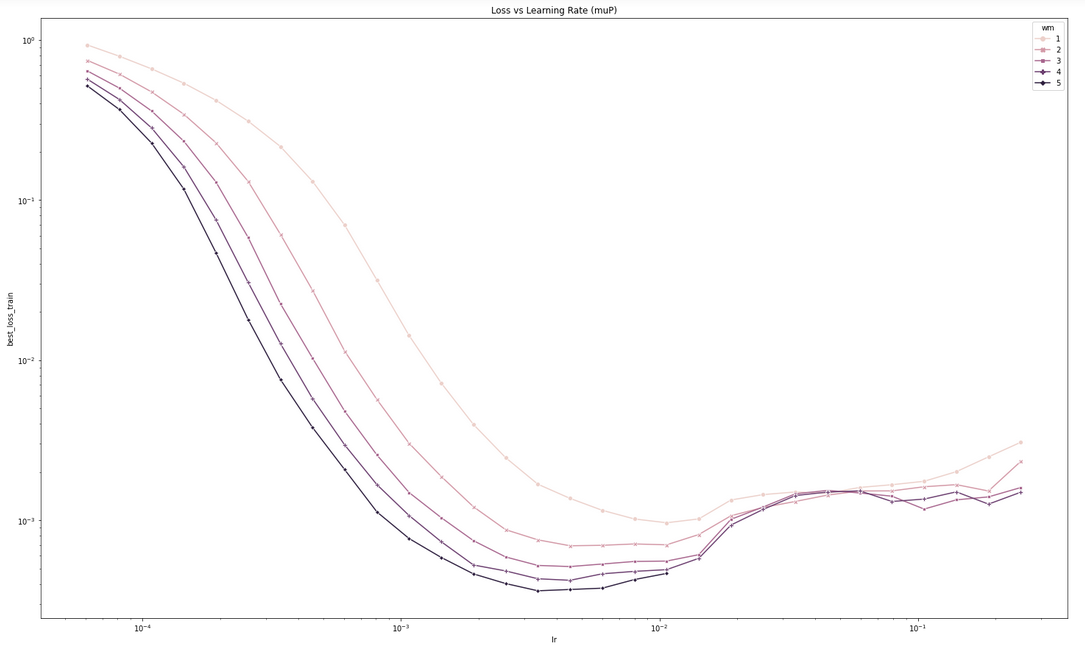Without muP :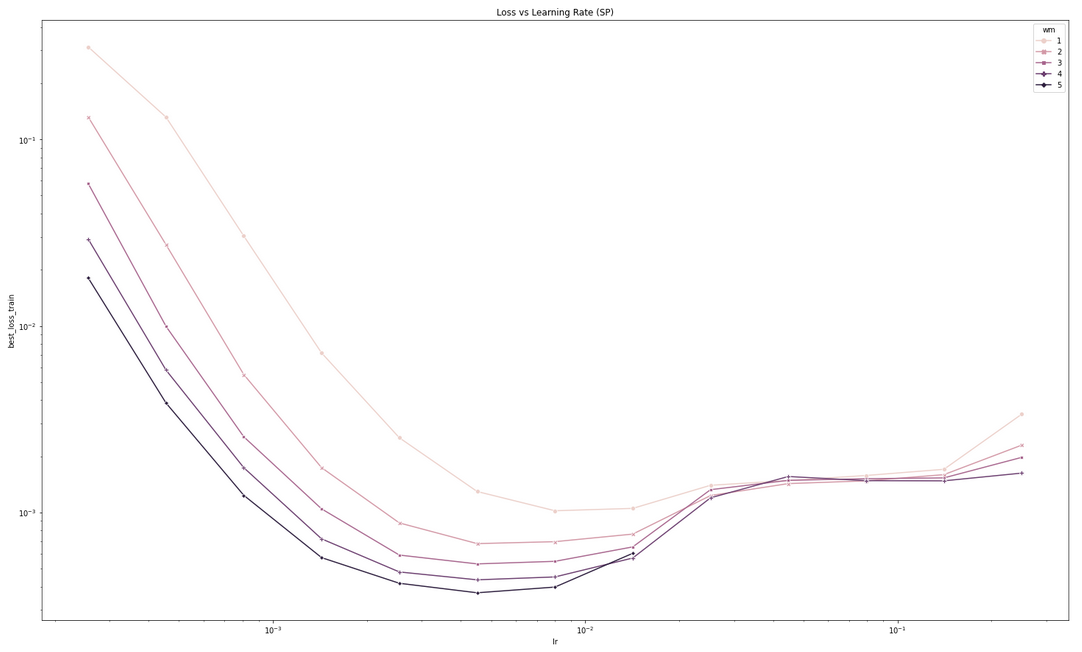As you can see on the two figures, there is no visible difference between the two scenarios: In both case, minima are aligned except for those with wm=1 Do you have an idea why it is happening ? Thanks for your help

opened by NicolasWinckler 5
• #### Are parameters with no "infinite" dimensions allowed?

Hi,

Is it valid to have parameters that have no "infinite" dimensions? This line suggests that it is, but I can't find anything in the paper that explains how this case should be dealt with.

With thanks, Callum

opened by callumm-graphcore 5
• #### mu parametrization for channel attention

Hi, I have another question about the mu parametrization for a special attention mechanism - channel attention.

In standard scaled dot-product attention (also regarded as spatial attention), we have Q, K, V with shape `n x d` (ignoring heads) and we will calculate `softmax(scale * Q K^T) V` to get a `n x d` output, where `scale = 1/sqrt(d)` in SP and `scale = 1/d` in muP (or `1/sqrt(d_0) / width_mult` in muP for backward compatiblity).

In channel attention, we still have Q, K, V with shape `n x d` (ignoring heads). The different part is, we will calculate `(softmax(scale * Q^T K) V^T)^T` to get a `n x d` output, where `scale = 1/sqrt(n)` in SP. Since the attention map `Q^T K` now has shape `d x d` instead of `n x n`, I am not sure how the scale should be modified in SP accordingly. Should we use `1/sqrt(n) / width_mult`?

In addition, Appendix B - Matrix-Like, Vector-Like, Scalar-Like Parameters has some interpretation behind the scale:

a multiplier of order 1=fan_in should accompany any weight that maps an infinite dimension to a finite one. This interpretation then nicely covers both the output logits and the attention logits (i.e. 1/d attention).

But such interpretation may not be directly used as a guidance to set up the scale in the channel attention.

Thanks!

opened by xwjabc 5

Hi, thank you for your great project for hyperparameter tuning!

As our team migrating the mup to other training framework, it occurs to us that the `MuAdam` does not scale the learning rate for output weights as the TP5 paper illustrated: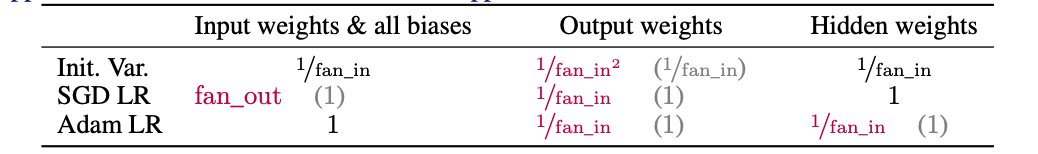https://github.com/microsoft/mup/blob/c9d67001c47ae254ea4b7e26146ffd059520b6ba/mup/optim.py#L55-L70

It seems to us that only the lr of hidden layer (the layer with 2 inf dimensions) is scaled w.r.t fanin, but the output weight is ignored. We wonder if this is intended. Thank you!

opened by zhuzilin 4
• #### Multiple nn.Linear layers

Hi, Your project is really interesting, so I am learning how to apply it to some specific models. For example, the model has multiple nn.Linear layers like in wav2vec 2.0 (self.post_extract_proj, self.project_q, self.project_inp, self.target_glu, self.final_proj), should I replace all these layers to MuReadout?

``````class Wav2Vec2Model(BaseFairseqModel):
def __init__(self, cfg: Wav2Vec2Config):
super().__init__()
self.cfg = cfg

feature_enc_layers = eval(cfg.conv_feature_layers)
self.embed = feature_enc_layers[-1]

self.feature_extractor = ConvFeatureExtractionModel(
conv_layers=feature_enc_layers,
dropout=0.0,
mode=cfg.extractor_mode,
conv_bias=cfg.conv_bias,
)

self.post_extract_proj = (
nn.Linear(self.embed, cfg.encoder_embed_dim)
if self.embed != cfg.encoder_embed_dim and not cfg.quantize_input
else None
)

self.dropout_input = nn.Dropout(cfg.dropout_input)
self.dropout_features = nn.Dropout(cfg.dropout_features)

self.quantizer = None
self.input_quantizer = None

self.n_negatives = cfg.num_negatives
self.cross_sample_negatives = cfg.cross_sample_negatives
self.codebook_negatives = cfg.codebook_negatives
self.negatives_from_everywhere = cfg.negatives_from_everywhere

self.logit_temp = cfg.logit_temp

final_dim = cfg.final_dim if cfg.final_dim > 0 else cfg.encoder_embed_dim

if cfg.quantize_targets:
vq_dim = cfg.latent_dim if cfg.latent_dim > 0 else final_dim
self.quantizer = GumbelVectorQuantizer(
dim=self.embed,
num_vars=cfg.latent_vars,
temp=cfg.latent_temp,
groups=cfg.latent_groups,
combine_groups=False,
vq_dim=vq_dim,
time_first=True,
weight_proj_depth=cfg.quantizer_depth,
weight_proj_factor=cfg.quantizer_factor,
)
self.project_q = nn.Linear(vq_dim, final_dim)
else:
self.project_q = nn.Linear(self.embed, final_dim)

if cfg.quantize_input:
if cfg.same_quantizer and self.quantizer is not None:
vq_dim = final_dim
self.input_quantizer = self.quantizer
else:
vq_dim = cfg.latent_dim if cfg.latent_dim > 0 else cfg.encoder_embed_dim
self.input_quantizer = GumbelVectorQuantizer(
dim=self.embed,
num_vars=cfg.latent_vars,
temp=cfg.latent_temp,
groups=cfg.latent_groups,
combine_groups=False,
vq_dim=vq_dim,
time_first=True,
weight_proj_depth=cfg.quantizer_depth,
weight_proj_factor=cfg.quantizer_factor,
)
self.project_inp = nn.Linear(vq_dim, cfg.encoder_embed_dim)

torch.FloatTensor(cfg.encoder_embed_dim).uniform_()
)

self.encoder = TransformerEncoder(cfg)
self.layer_norm = LayerNorm(self.embed)

self.target_glu = None
if cfg.target_glu:
self.target_glu = nn.Sequential(
nn.Linear(final_dim, final_dim * 2), nn.GLU()
)

self.final_proj = nn.Linear(cfg.encoder_embed_dim, final_dim)
``````

Thank you! ^^

opened by windspirit95 4
• #### Consider decoupled weight decay optimizers?

Hi! I'm a big fan of this project and I noticed that MUP has some wrappers for common optimizers `MuAdam`, `MuAdamW`, and `MuSGD`. Given the focus on hyperparameter stability and tuning, I was wondering if you might be interested in adding / experimenting with the decoupled weight decay optimizers from this paper (https://arxiv.org/abs/1711.05101)?

For context, PyTorch's implementations of `SGD`, `Adam`, and even `AdamW` all scale the weight decay by the learning rate in their update step, and I've found that this makes it tough to tune the two values independently (if you increase LR, you also silently increase the effective WD). This is due to PyTorch's scheduler implementation which only updates an Optimizer's LR, and so they schedule the WD in sync by multiplying the two values together.

E.g. here is Pytorch's AdamW code, which shows why it is not really decoupled: https://github.com/pytorch/pytorch/blob/11231b0f935c092598c994a4bab686485aac1856/torch/optim/adamw.py#L248

The "correct" way to decouple LR, WD is described in the paper, and we have some PyTorch-ready implementations here (code, docs) in MosaicML's Composer library. Though I haven't seen any examples in MUP yet of tuning the WD across model sizes, I feel like this could be a common hparam that users want to tune and that `DecoupledSGDW` or `DecoupledAdamW` could help make it more stable :)

opened by abhi-mosaic 4
• #### Finetuning a Pretrained Model Using MuP

Somewhat of a naive question, but say we have pretrained a model and now want to finetune it on a downstream task. Is there any reason we shouldn't replace the MuP layers with the equivalent `torch` layers? I have to imagine that we don't need to use `MuP` here, but want to make sure that this doesn't break anything if we replace them

opened by zanussbaum 3
• #### Batch size, Seq len, Step Transfering

Hi! I didn't fully understand how the transfer of parameters such as batch_size/seq_len/steps should work (Figure 17, 19 in the article). Also I didn't find any mention of this either in the article or in the library code It would seem that according to the idea of mup, we shouldn't do any scales for these parameters, but then it is unclear how it works with batch size. Should I forget about all lr/batch_size dependency rules? what will happen to the convergence rate in this case ?

opened by timothyxp 2
• #### Coord check looks good, but μTransfer is not working as expected

Hello, μP team! Very excited to see you open source your excellent work! I was looking to apply μP on our work, and on Megatron-DeepSpeed I modified the training script as suggested in the tutorial, set the infshape, reset parameters initialization, put on MuAdam, and got a coord_check that looked successful. But when we transfer the learning rate that performed well on the 350M GPT model to the large model 1.3B, we found that the 1.3B could not withstand such a large learning rate and eventually produced NaN.

I was wondering what details might not have been taken into account, or the conditions were not met, causing μTransfer to fail. How should I debug, or μTransfer just won't work under this condition?

The following is the experimental information.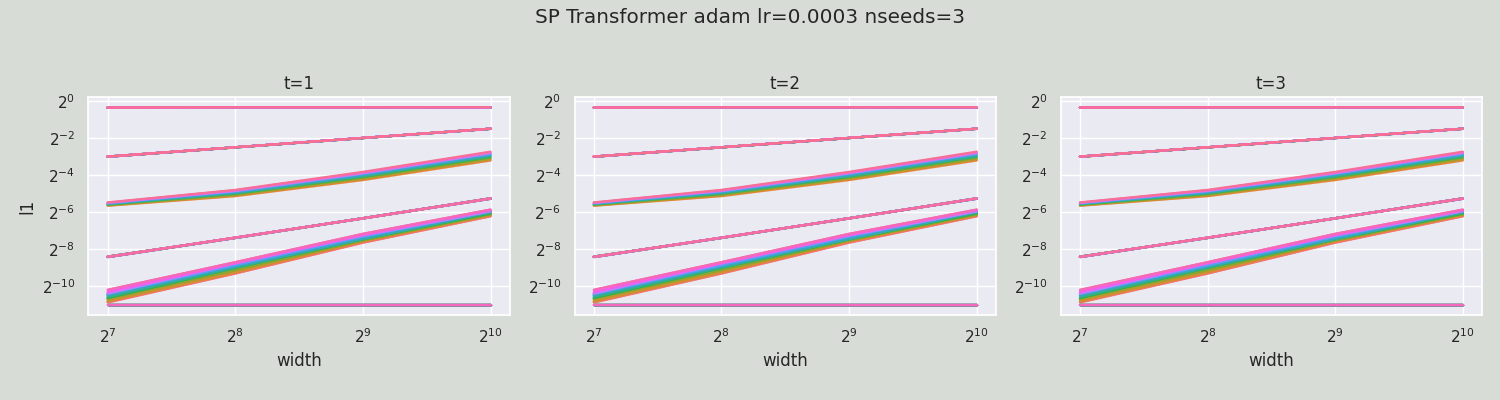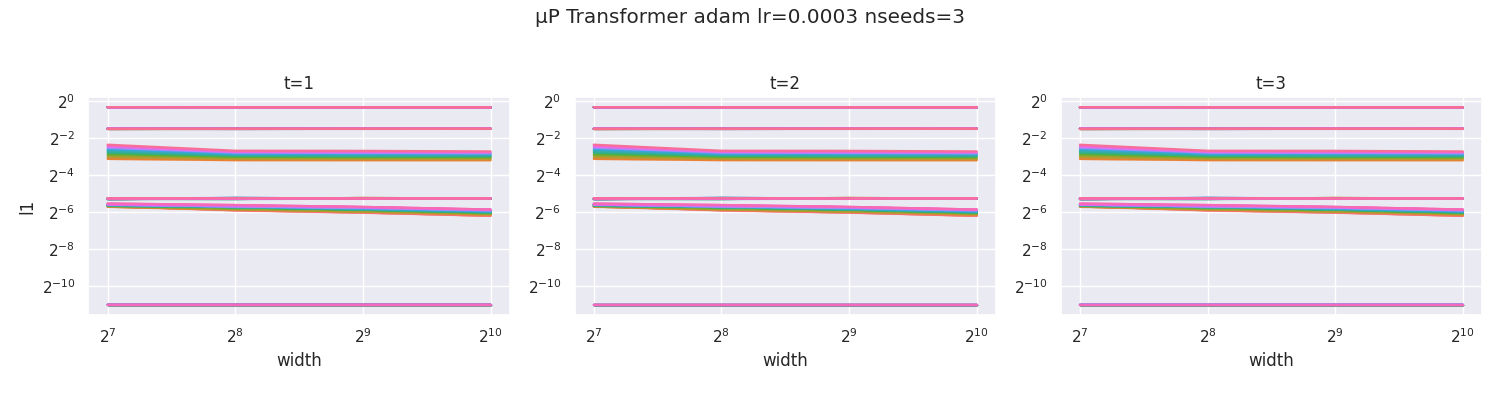350M -> 1.3B GPT model μTransfer training loss( tensorborad link ):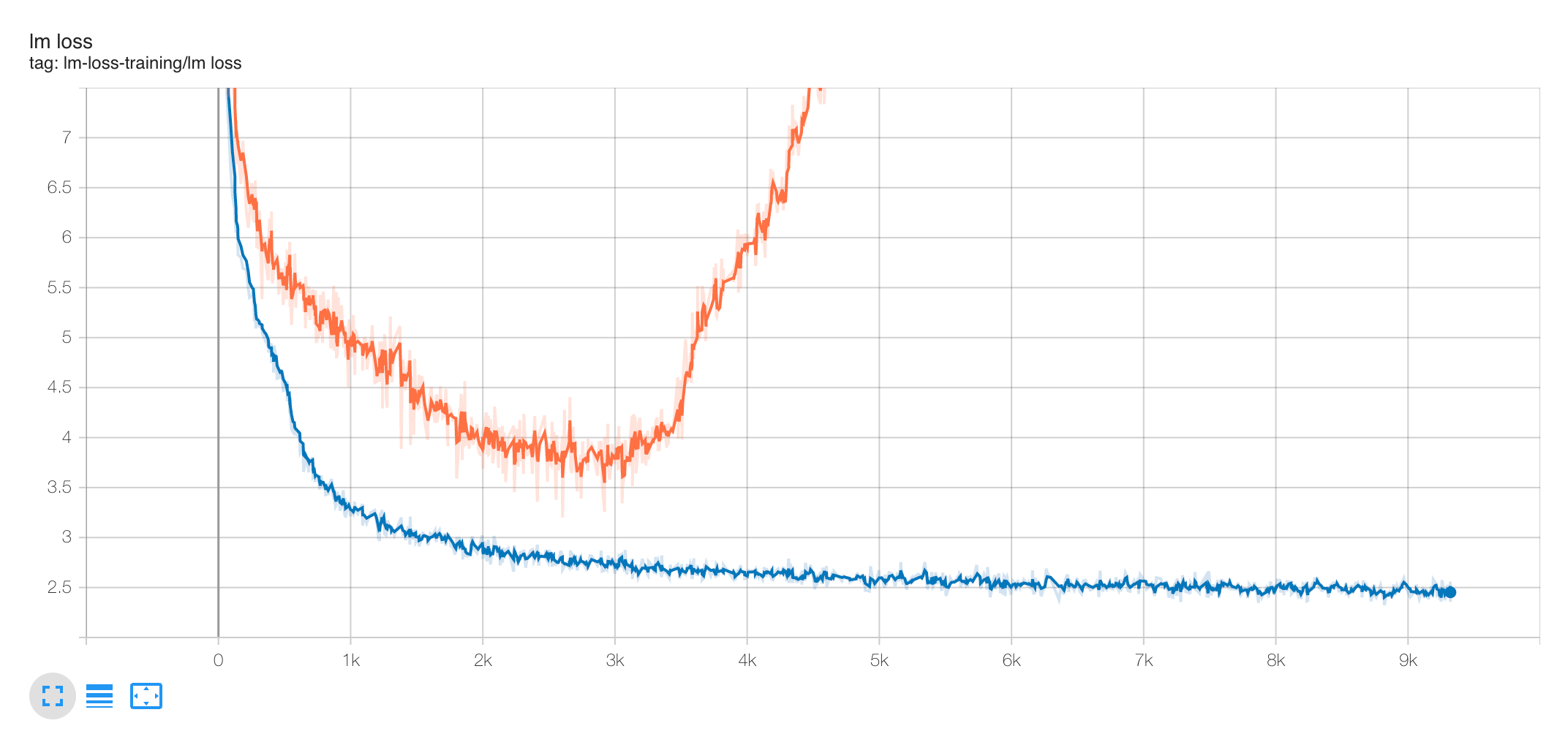I think it may be a bit redundant, but if you are interested, the transformation of μP is here:

1. Replace output layer with MuReadout, https://github.com/shjwudp/Megatron-LM/blob/mup/megatron/model/gpt_model.py#L250
2. Make sure to use 1/d instead of 1/sqrt(d) attention scaling, https://github.com/shjwudp/Megatron-LM/blob/mup/megatron/model/transformer.py#L175
3. Set infshape and do mup parameter initiliaze, https://github.com/shjwudp/Megatron-LM/blob/mup/pretrain_gpt.py#L110
5. Implement the equivalent `MuReadout._rescale_parameters` operation, https://github.com/shjwudp/Megatron-LM/blob/mup/megatron/mpu/layers.py#L191
6. Modify lr scheduler to update lr according to width, https://github.com/shjwudp/Megatron-LM/blob/mup/megatron/learning_rates.py#L127
7. Coord check, https://github.com/shjwudp/Megatron-LM/blob/mup/megatron/mup_utils.py#L16
opened by shjwudp 4
• #### Does mup support Swin Transformer v2 model?

Hi, we are trying to use mup tool to tune Swin Transformer v2 model. I modified the code of Swin Transformer v2 to adapt mup and executed the "save base shape" and "coordinate check". The results of "coordinate check" shows that it can not meet the requirements of mup.

Does mup support the Swin Transformer v2 model?

For the code of "swin_transformer_v2.py", I modified the following code （Because Swin Transformer v2 doesn't use "1/sqrt(d) attention scaling", I don't modify it）:

1. replaced the output layper nn.Linear with MuReadout
2. replaced std normal init with mup normal init
``````self.norm = norm_layer(self.num_features)
# self.head = nn.Linear(self.num_features, num_classes) if num_classes > 0 else nn.Identity()
### muP: replace nn.Linear with MuReadout

self.apply(self._init_weights)
for bly in self.layers:
bly._init_respostnorm()
``````
``````def _init_weights(self, m, readout_zero_init=False, query_zero_init=False):
### muP: swap constant std normal init with normal_ from `mup.init`.
### Because `_init_weights` is called in `__init__`, before `infshape` is set,
### we need to manually call `self.apply(self._init_weights)` after calling
### `set_base_shape(model, base)`
if isinstance(m, nn.Linear):
m.weight.data.zero_()
else:
if hasattr(m.weight, 'infshape'):
normal_(m.weight, mean=0.0, std=.02)
else:
trunc_normal_(m.weight, std=.02)
if isinstance(m, nn.Linear) and m.bias is not None:
nn.init.constant_(m.bias, 0)
### End muP
elif isinstance(m, nn.LayerNorm):
nn.init.constant_(m.bias, 0)
nn.init.constant_(m.weight, 1.0)
``````

For the code of "main.py" of Swin Transformer, I added "save base shape" and "coordinate check" functions.

``````def main(config, args):

logger.info(f"Creating model:{config.MODEL.TYPE}/{config.MODEL.NAME}")
model = build_model(config)
logger.info(str(model))

### muP
if args.save_base_shapes:
print(f'saving base shapes at {args.save_base_shapes}')
base_shapes = get_shapes(model)
delta_config = copy.deepcopy(config)
delta_config.defrost()
delta_config.MODEL.SWINV2.EMBED_DIM *= 2  # Modify SwinV2 embed dim
delta_config.MODEL.SWIN.EMBED_DIM *= 2  # Modify Swin embed dim
# delta_config.MODEL.SWIN_MOE.EMBED_DIM *= 2  # Modify Swin_moe embed dim
delta_config.MODEL.SWIN_MLP.EMBED_DIM *= 2  # Modify Swin_mlp embed dim

delta_shapes = get_shapes(
# just need to change whatever dimension(s) we are scaling
build_model(delta_config)
)
make_base_shapes(base_shapes, delta_shapes, savefile=args.save_base_shapes)
print('done and exit')
import sys;
sys.exit()
print('done')
else:
print(f'using own shapes')
set_base_shapes(model, None)
print('done')
``````
``````### muP
def coord_check(mup, config, lr, optimizer, nsteps, nseeds, args, plotdir='', legend=False):

def gen(w, standparam=False):
def f():
delta_config = copy.deepcopy(config)
delta_config.defrost()
delta_config.MODEL.SWINV2.EMBED_DIM = w  # Modify SwinV2 embed dim
delta_config.MODEL.SWIN.EMBED_DIM = w  # Modify Swin embed dim
# delta_config.MODEL.SWIN_MOE.EMBED_DIM = w  # Modify Swin_moe embed dim
delta_config.MODEL.SWIN_MLP.EMBED_DIM = w  # Modify Swin_mlp embed dim
model = build_model(delta_config)

if standparam:
set_base_shapes(model, None)
else:
return model
return f

optimizer = optimizer.replace('mu', '')
widths = (12, 24, 48, 96, 192)
models = {w: gen(w, standparam=not mup) for w in widths}

# train_data = batchify(corpus.train, batch_size, device=args.device)
df = get_coord_data(models, data_loader_train, mup=mup, lr=lr, optimizer=optimizer, flatten_output=True,
nseeds=nseeds, nsteps=nsteps, lossfn='xent')

prm = 'muP' if mup else 'SP'
return plot_coord_data(df, legend=legend,
save_to=os.path.join(plotdir, f'{prm.lower()}_trsfmr_{optimizer}_coord.png'),
suptitle=f'{prm} Transformer {optimizer} lr={lr} nseeds={nseeds}',
face_color='xkcd:light grey' if not mup else None)
``````
``````if __name__ == '__main__':
args, config = parse_option()

......

### muP
if args.coord_check:
print('testing parametrization')
import os
os.makedirs('coord_checks', exist_ok=True)
plotdir = 'coord_checks'
nsteps=args.coord_check_nsteps, nseeds=args.coord_check_nseeds, args=args,
plotdir=plotdir, legend=False)
nsteps=args.coord_check_nsteps, nseeds=args.coord_check_nseeds, args=args,
plotdir=plotdir, legend=False)
import sys
sys.exit()

main(config, args)
``````

The results of "coordinate check" show that there is only a small difference between "mup" and "SP". sorry, I can't upload pictures. Could you please help us to check if mup can support Swin Transformer v2 model? or there are some other reasons? Thanks a lot.

opened by shiyf129 1
• #### integration with Flax?

Is there any interest in integrating this work with Flax?

They already have a init function, decoupling parameters initialization from model definition which could make introducing mup fairly plug-and-play.

Plus they relie on optax for their optimizers. As that library has a focus on composability, you might be able to introduce a transformation that takes an optimizer and makes it mup compatible.

Overall, I believe the Flax ecosystem could make mup more easily accessible to people.

opened by nestordemeure 4
##### Releases(v1.0.0)
###### Microsoft
Open source projects and samples from Microsoft###### A script helps the user to update Linux and Mac systems through the terminal

Description This script helps the user to update Linux and Mac systems through the terminal. All the user has to install some requirements and then ru

2 Jan 23, 2022
###### Jurigged lets you update your code while it runs.

jurigged Jurigged lets you update your code while it runs. Using it is trivial: python -m jurigged your_script.py Change some function or method with

767 Dec 28, 2022
###### Update of uncaptcha2 from 2019

YouTube Video Proof of Concept I created a new YouTube Video with technical Explanation for breaking Google's Audio reCAPTCHAs: Click on the image bel

153 Dec 20, 2022
###### A simple tool to update bib entries with their official information (e.g., DBLP or the ACL anthology).

Rebiber: A tool for normalizing bibtex with official info. We often cite papers using their arXiv versions without noting that they are already PUBLIS

2k Jan 1, 2023
###### Retrieve information from DBLP and update BibTex files automatically

Rebib TLDR: This script retrieves information from DBLP to update your BibTex files. python rebib.py --bibfile xxx.bib It first parses the bib entries

49 Jan 1, 2023
###### Package, distribute, and update any app for Linux and IoT.

Snapcraft Package, distribute, and update any app for Linux and IoT. Snaps are containerised software packages that are simple to create and install.

1.1k Jan 2, 2023
###### A script to automatically update bot status at GitHub as well as in Telegram channel.

Support BotStatus ~ A simple & short repository to show your bot's status in your GitHub README.md file as well as in you channel. ⚠️ This repo should

55 Dec 13, 2022
###### A script to automatically update bot status at GitHub as well as in Telegram channel.

A simple & short repository to show your bot's status in your GitHub README.md file as well as in you channel.

55 Dec 13, 2022
###### Code I use to automatically update my videos' metadata on YouTube

mCodingYouTube This repository contains the code I use to automatically update my videos' metadata on YouTube, including: titles, descriptions, tags,

19 Oct 7, 2022
###### A script for performing OTA update over BLE on ESP32

A script for performing OTA update over BLE on ESP32

18 Dec 15, 2022
###### lightweight, fast and robust columnar dataframe for data analytics with online update

streamdf Streamdf is a lightweight data frame library built on top of the dictionary of numpy array, developed for Kaggle's time-series code competiti

23 May 19, 2022
###### A python module to update the console without flashing.

A python module to update the console without flashing.

112 Dec 19, 2022
###### A Python script to update Spotify Playlist data every 5 minutes.

Spotify Playlist Updater A Python script to update Spotify Playlist data every 5 minutes. Description An automatic playlist updater using Spotify API

6 Nov 24, 2022
###### An automatic django's update checker and MS teams notifier

Django Update Checker This is small script for checking any new updates/bugfixes/security fixes released in django News & Events and sending correspon

4 Sep 26, 2022
###### program to store and update pokemons using SQL and Flask

Pokemon SQL and Flask Pokemons api in python. Technologies flask pymysql Description PokeCorp is a company that tracks pokemon and their trainers arou

1 Oct 20, 2021
###### Backup a folder to an another folder by using mirror update method.

Mirror Update Backup Backup a folder to an another folder by using mirror update method. How to use Install requirement pip install -r requirements.tx

1 Nov 21, 2022
###### Online-update est un programme python permettant de mettre a jour des dossier et de fichier depuis une adresse web.

Démarrage rapide Online-update est un programme python permettant de mettre a jour des dossier et de fichier depuis une adresse web. Mode préconfiguré

2 Nov 26, 2021
###### This Lambda will Pull propagated routes from TGW and update VPC route table

AWS-Transitgateway-Route-Propagation This Lambda will Pull propagated routes from TGW and update VPC route table. Tested on python 3.8 Lambda AWS INST

4 Jan 20, 2022
###### Easily update resume to naukri with one click

NAUKRI RESUME AUTO UPDATER I am using poetry for dependencies. you can check or change in data.txt file for username and password Resume file must be

1 May 2, 2022
###### Install, run, and update apps without root and only in your home directory

Qube Apps Install, run, and update apps in the private storage of a Qube Building instrutions

26 Dec 27, 2022